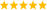# Biomedical User Group Discussions

cancel
Showing results for
Did you mean:

## RMS value of EMG calculated in LabView varies from the RMS value found using lab view.. For Example in LAb view i got RMS value as 79.85 whereas in MATLAB 0.089

RMS value of EMG calculated in LabView varies from the RMS value found using lab view.. For Example in LAb view i got RMS value as 79.85 whereas in MATLAB 0.089

Message 1 of 2
(1,116 Views)

## Re: RMS value of EMG calculated in LabView varies from the RMS value found using lab view.. For Example in LAb view i got RMS value as 79.85 whereas in MATLAB 0.089

We cannot help unless you show us your code. Most likely you are calculating wrong.

Since this is a plain LabVIEW questions, it would be better to discuss it in the LabVIEW forum.

(What is the difference between "LabView", "lab view" and "LAb view". I am only familiar with LabVIEW.)LabVIEW ChampionDo more with less code and in less timeMessage 2 of 2
(1,098 Views)